# Chassis Control¶

Chassis Control include drives MoonBot Kit Motor Module and driving of Encoder in Motor.

By calling these modules, the motor chassis can move.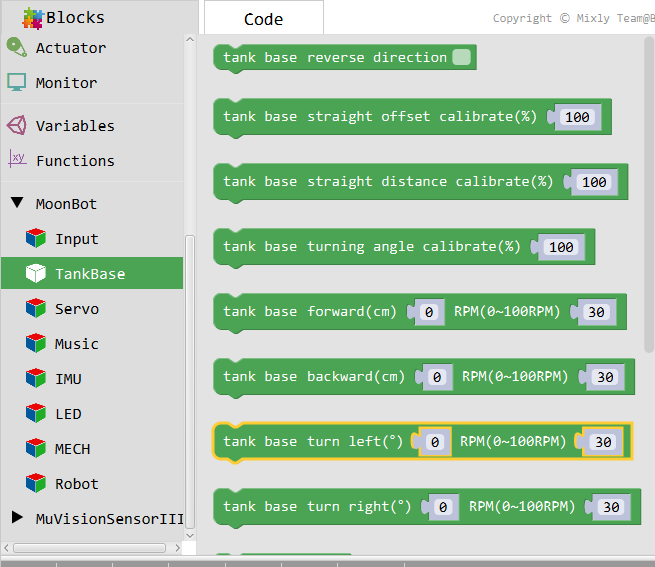## Reversal direction¶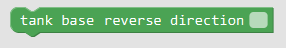Introduction

The direction of motion of the flip motor.

Parameters
Reversal direction
• `true` :Reversal direction

• `false` :Default direction

## Straight-line offset correction¶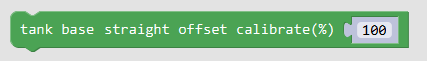Introduction

Because of friction, installation deviation and other disturbances, the chassis will be offset in a certain direction when it goes straight.

Direct migration caused by external disturbance can be corrected by `direct migration correction `module.

Introduction
Straight-line offset correction(%)
• `0~200` :>100 Correction to the right,<100 Correction to the left

## Straight Distance Correction¶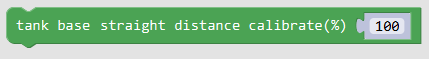Introduction

Because of friction, installation deviation and other disturbances, chassis traveling a certain distance will have the situation of inadequate direct travel.

Through the `direct distance correction `module, the situation of the out-of-place direct distance caused by external disturbance can be corrected.

Before correcting the straight-line distance, it is suggested that straight-line migration correction’be carried out first.`

Introduction
Straight Distance Correction(%)
• `0~+∞` :>100 Increased direct distance,<100 Straight distance decreases

## Turning Angle Correction¶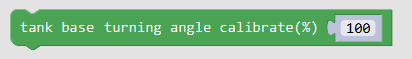Introduction

Because of friction, installation deviation and other disturbances, chassis rotating at a certain angle will have the situation that the turning angle is not in place.

Through the `turning angle correction’module, the situation that the turning angle caused by external disturbance is not in place can be corrected.

Before correcting the turning angle, it is suggested that `straight-line offset correction’ and `straight-line distance correction’ should be carried out first.

Introduction
Turning Angle Correction(%)
• `0~+∞` :>100 Increased turning angle,<100 Reduced turning angle

## Forward¶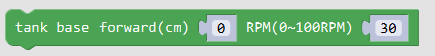Introduction

Control the chassis to move forward at a given speed until it stops at a given distance.

The module ** calls the encoder module ** to ensure that the corresponding encoder has been connected to the corresponding port

Parameters
Forward Distance(cm)
• `Distance value` :Given straight distance,Unit: cm

speed
• `Speed Value` :Given Speed of Direct Motor,Unit: RPM

## Backward¶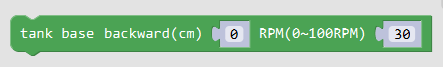Introduction

The control chassis runs backwards at a given speed until it stops at a given distance.

The module ** calls the encoder module ** to ensure that the corresponding encoder has been connected to the corresponding port.

Parameters
Backward distance(cm)
• `Distance value` :Given straight distance,Unit: cm

speed
• `Speed Value` :Given Speed of Direct Motor,Unit: RPM

## Left turn¶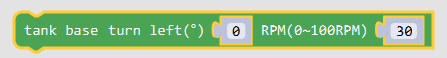Introduction

Control the chassis to turn left at a given speed to a given angle and stop.

The module ** calls the encoder module ** to ensure that the corresponding encoder has been connected to the corresponding port.

Parameters
Left turn angle(°)
• `Angle value` :Given a straight angle,Unit: °

speed
• `Speed Value`:Given Speed of Direct Motor,Unit: RPM

## Right turn¶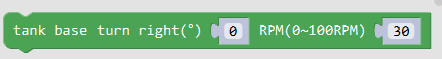Introduction

Control the chassis to turn right at a given speed to a given angle and stop.

The module ** calls the encoder module ** to ensure that the corresponding encoder has been connected to the corresponding port.

Parameters
Right turn angle(°)
• `Angle value` :Given a straight angle,Unit: °

speed
• `Speed Value` :Given Speed of Direct Motor,Unit: RPM

## Stop¶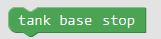Introduction

The chassis stops turning.

## Motor write-in value¶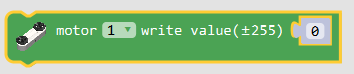Introduction

Write the analog to the motor at the corresponding port.

Parameters
Motor port
• `1` :Motor port 1

• `2` :Motor port 2

value
• `±255` :Write the value of the analog,>0 Turn Forward,<0 Turn back,=0 Stop turning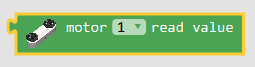Introduction

Read the analog value of the corresponding motor port.

Parameters
Motor port
• `1` :Motor port 1

• `2` :Motor port 2

Return
• `value` :Value of motor analogue

## Writing Speed of Motor¶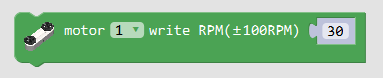Introduction

Write the speed to the motor at the corresponding port.

The module ** calls the encoder module ** to ensure that the corresponding encoder has been connected to the corresponding port.

Parameters
Motor port
• `1` :Motor port 1

• `2` :Motor port 2

value
• `±60` :Write the value of the analog,>0 Turn Forward,<0 Turn back,=0 Stop turning,unit:RPM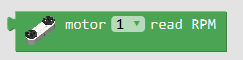Introduction

Read the speed of the corresponding motor port.

Parameters
Motor port
• `1` :Motor port 1

• `2` :Motor port 2

Return
• `speed` :motor speed ,unit:RPM